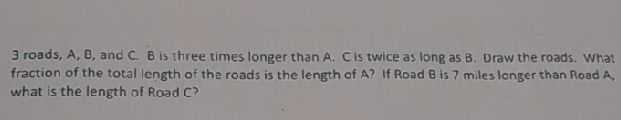### ¿Todavía tienes preguntas de matemáticas?

Pregunte a nuestros tutores expertos
Algebra
Pregunta3 roads, $$A , B ,$$ and $$C B$$ is three times longer than $$A$$ . $$C$$ is twice as long as $$B$$ . Draw the roads. What fraction of the total length of the roads is the length of $$A$$ ? If Road $$B$$ is $$7$$ miles longer than Road $$A$$ . what is the length of Road $$C$$ ?